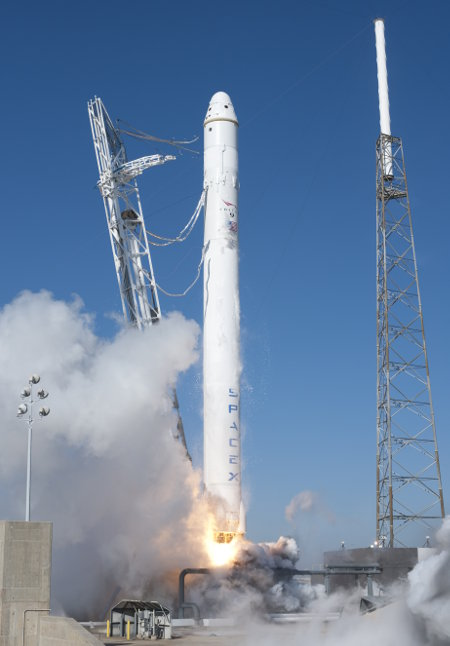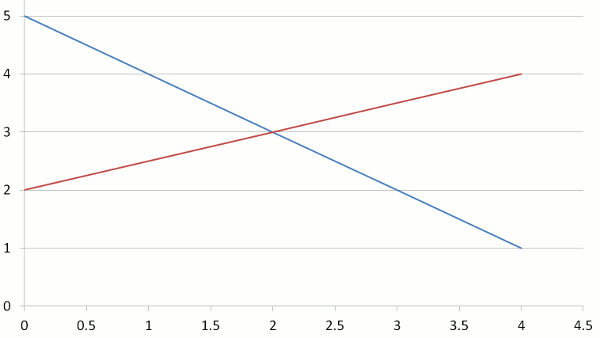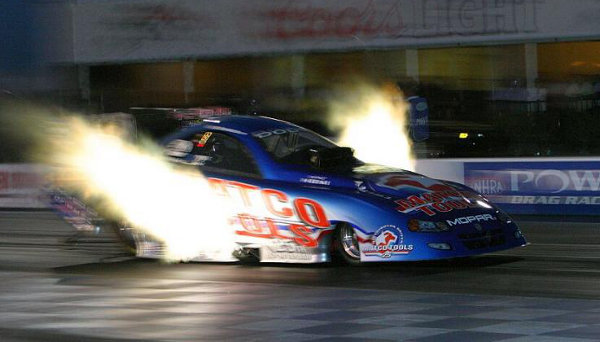# Solve Simultaneous Equations By GraphingIn 2012, SpaceX successfully launched the world's first privately-owned rocket to the International Space Station which orbits 220 miles (350 kilometres) above the Earth. The path of the rocket and the path of the ISS intersected at one point in space. The mathematics to do this is called solving the equations simultaneously.

Here, we will graph 2 linear equations and identify the point of intersection at which the 2 graphed lines meet. At this point, the equations have the same x-value and y-value.

## Example One - Solving Two Linear Equations

Solve the equations y = –x + 5 and y = 12 x + 2Point of intersection = ( 2 , 3 )
The simultaneous solution for both equations is x = 2 and y = 3.

## Example Two - Drag Car OvertakingA fast drag car gives a head start of 2 metres to a slow drag car, but still overtakes it.
If x is the time in seconds and y is the distance travelled in metres,

• the equation for the fast car is y = 4x
• the equation for the slow car is y = 3x + 2

At what point (x,y) or (time, distance) does the fast car overtake the slow car?

Fast car (blue line in graph)

 x 0 1 2 3 y = 4x 0 4 8 12

Slow car (red line in graph)

 x 0 1 2 3 y = 3x + 2 2 5 8 11The point of intersection is (2,8).
This means that the fast car overtakes the slow car after 2 seconds and at 8 metres from the start.